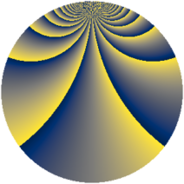# Properties

 Label 2760.2.deLevel $2760$ Weight $2$ Character orbit 2760.de Rep. character $\chi_{2760}(77,\cdot)$ Character field $\Q(\zeta_{44})$ Dimension $11360$ Sturm bound $1152$

# Related objects

## Defining parameters

 Level: $$N$$ $$=$$ $$2760 = 2^{3} \cdot 3 \cdot 5 \cdot 23$$ Weight: $$k$$ $$=$$ $$2$$ Character orbit: $$[\chi]$$ $$=$$ 2760.de (of order $$44$$ and degree $$20$$) Character conductor: $$\operatorname{cond}(\chi)$$ $$=$$ $$2760$$ Character field: $$\Q(\zeta_{44})$$ Sturm bound: $$1152$$

## Dimensions

The following table gives the dimensions of various subspaces of $$M_{2}(2760, [\chi])$$.

Total New Old
Modular forms 11680 11680 0
Cusp forms 11360 11360 0
Eisenstein series 320 320 0

## Trace form

 $$11360q - 36q^{6} - 72q^{7} + O(q^{10})$$ $$11360q - 36q^{6} - 72q^{7} - 36q^{10} - 6q^{12} - 36q^{15} - 80q^{16} - 10q^{18} - 64q^{22} - 72q^{25} - 100q^{28} + 2q^{30} - 112q^{31} - 12q^{33} - 68q^{36} - 76q^{40} + 2q^{42} - 112q^{46} - 82q^{48} + 176q^{52} + 8q^{55} - 12q^{57} - 84q^{58} - 34q^{60} - 92q^{63} + 12q^{66} - 160q^{70} + 14q^{72} - 72q^{73} + 40q^{76} - 74q^{78} - 40q^{81} - 92q^{82} - 60q^{87} + 28q^{88} + 288q^{90} - 36q^{96} - 72q^{97} + O(q^{100})$$

## Decomposition of $$S_{2}^{\mathrm{new}}(2760, [\chi])$$ into newform subspaces

The newforms in this space have not yet been added to the LMFDB.